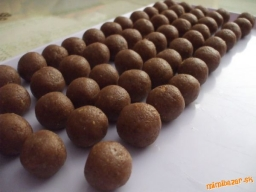# Bullets 7405

Pavol, Igor, and Kubo played bullets. They had a total of 25 marbles. Palo had 6 more bullets than Kubo at the start. Then Igor won 8 bullets from Pavol, and thus Igor had the same number of bullets as Kubo. How many marbles does Pal have left?

x =  7

### Step-by-step explanation:

p+i+k = 25
p = 6+k
i+8 = k
x = p-8

i+k+p = 25
k-p = -6
i-k = -8
p-x = 8

Row 3 - Row 1 → Row 3
i+k+p = 25
k-p = -6
-2k-p = -33
p-x = 8

Pivot: Row 2 ↔ Row 3
i+k+p = 25
-2k-p = -33
k-p = -6
p-x = 8

Row 3 - 1/-2 · Row 2 → Row 3
i+k+p = 25
-2k-p = -33
-1.5p = -22.5
p-x = 8

Row 4 - 1/-1.5 · Row 3 → Row 4
i+k+p = 25
-2k-p = -33
-1.5p = -22.5
-x = -7

x = -7/-1 = 7
p = -22.5/-1.5 = 15
k = -33+p/-2 = -33+15/-2 = 9
i = 25-k-p = 25-9-15 = 1

i = 1
k = 9
p = 15
x = 7

Our linear equations calculator calculates it.Did you find an error or inaccuracy? Feel free to write us. Thank you!

Tips for related online calculators
Do you have a system of equations and looking for calculator system of linear equations?